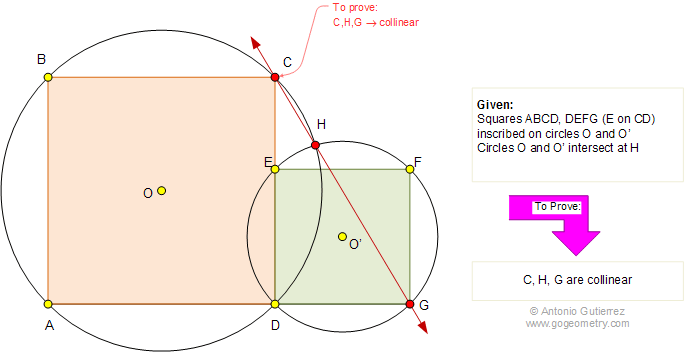# Problem 723: Squares, Circumscribed Circles, Collinearity. High School, College

In the figure below, the squares ABCD and DEFG are inscribed in the circles O and O', respectively, Point E is on CD and circles O and O' intersect at H. Prove that C, H, G are collinear.## More Geometry Problems

Geometry Problem 722.
Squares, Circumscribed Circles, Collinear points.

Geometry Problem 721.
Triangle, Orthocenter, Circumcircle, Altitude, Collinear points.

Geometry Problem 720.
Excenter, Intersecting Circles, Angle, Measurement.

Home | Geometry | Search | Problems | Square | Circles | Intersecting Circles | Collinear Points | Open Problems | All Problems | Visual Index | 721-730 | Email | Post a solution/comment | By Antonio Gutierrez